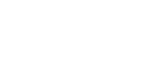ISSN 2542–0380 Труды Института механики им. Р.Р. Мавлютова Электронный научный журнал | Electronic Scientific Journal Proceedings of the Mavlyutov Institute of Mechanics© Институт механики
им. Р.Р. Мавлютова
УФИЦ РАНDelev V.A., Skaldin O.A., Timirov Y.I. The method for determination of flexoelectric coefficients of nematic liquid crystals. Proceedings of the Mavlyutov Institute of Mechanics. 2017. 12(1). 101–108.
2017. Vol. 12. Issue 1, Pp. 101–108
URL: http://proc.uimech.org/uim2017.1.015,en
DOI: 10.21662/uim2017.1.015
The method for determination of flexoelectric coefficients of nematic liquid crystals
Delev V.A., Skaldin O.A., Timirov Y.I.
Institute of Molecule and Crystal Physics, Ufa

### Abstract

A method for determining the flexoelectric coefficients of a nematic liquid crystal (NLC) is proposed. The principle of method suggested is the measurement of characteristics of flexoelectric domains arising in the a polarization-optical microscope (electro-optical method). The digitized images of these domains is used for the optical contrast K determination. Linear fitting of the contrast K depending on the applied voltage U in vicinity of the threshold of flexoelectric domain formation allows rather precisely to determine the critical voltage Uc. The spatial period of the observed domains is found by means of a two-dimensional Fourier transformation of digitized images. Using the material parameters of the NLC in the linear analysis of the basic state stability, and the difference between the flexoelectric coefficients (e1–e3) as the fitting parameter, the threshold characteristics (critical voltage and characteristic spatial period) of the flexoelectric domains are calculated so that they were close to experimental ones. The flexocoefficient difference chosen in this way is accepted as the wanted result.

Keywords

liquid crystals,
flexoelectric effect,
flexoelectric instability,
flexoelectric domains,
flexoelectric coefficients

## Article outline

Problem: Determination of a difference of flexoelectric coefficients of a nematic liquid crystal (NLC) N-4-methoxybenzylidene-4-butylaniline (MBBA).

Methods and approaches: An electrooptical method was used for observation of the flexoelectric domains in NLC. The spatio-periodic structures, arising under an applied voltage, were observed by a polarized microscope AxioImager (Carl Zeiss). Obtained images were registered by means of the CCD camera with resolution of 512x512 points, frequency of 25 Hz and 256 gray levels of color. The digitized images were processed on the computer and the contrast of obtained images was calculated. The linear fitting of contrast K dependence on applied voltage U near the threshold allows to determine rather precisely the critical voltage Uc of spatio-periodic structure formation. To find the spatial period of observed domain structures the two-dimensional Fourier transformation was applied. Using the NLC material parameters in the linear analysis of stability of the basic state and the difference of flexoelectric coefficients (e1–e3) as fitting parameter, the threshold characteristics close to the experimental ones was obtained.

Choosing difference of flexoelectric coefficients was accepted as required result.